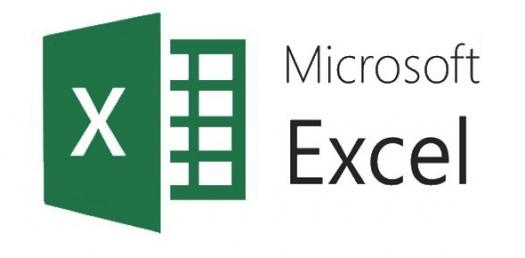# Basic Microsoft Excel Knowledge Quiz! Trivia

15 Questions | Total Attempts: 2468SettingsHow confident are you with your basic Microsoft Excel Knowledge? For this quiz, you will want to know which of the choices go with Block of Cells, which of the following is a cell reference, whether a label is the same as value, what is a cell reference, and what do you call boxes on a spreadsheet. This quiz is all about basic Microsoft Excel knowledge. You will have a great experience with this quiz.

• 1.
Which of the following definitions go with Block of Cells?
• A.

The cell with a black line around it showing where data will be added to the spreadsheet

• B.

A group of cells that are adjacent to each other and active

• C.

A group of cells adjacent to each other

• D.

A group of cells collected using the Ctrl key

• 2.
Which of the following is a cell reference?
• A.

C15

• B.

5C

• C.

CC

• D.

65,481

• 3.
A label is the same as a value.
• A.

True

• B.

False

• 4.
If you have a value in cell K6, what do you have in the cell?
• A.

Letters

• B.

Words

• C.

Sentences

• D.

Numbers

• 5.
A cell reference is the number of a row then the letter of the column.
• A.

True

• B.

False

• 6.
The file created in Excel is known as a workbook.
• A.

True

• B.

False

• 7.
A file in Excel is made up of 3 ______________ .
• A.

Rows

• B.

Columns

• C.

Worksheets

• D.

Workbooks

• 8.
In Microsoft Excel 2010, Where can you find the open, save, and print commands?
• A.

Home Ribbon

• B.

File Tab

• C.

Workbook Layout Button

• D.

Page Layout Button

• 9.
What do we call the boxes on a spreadsheet?
• A.

Formula bars

• B.

Grids

• C.

Home Boxes

• D.

Cells

• 10.
A _______ is a line of boxes going from left to right.
• A.

Row

• B.

Column

• C.

Formula String

• D.

• 11.
Which of the following formulas could you use to add several cells together?
• A.

=A1*B1*C1

• B.

A1+B1+C1=D1

• C.

=A1+B1+C1

• D.

A1*B1*C1

• 12.
Which of the following formulas could you use to add a range of cells together?
• A.

SUM()

• B.

=(SUM)

• C.

=+SUM()

• D.

=SUM()

• 13.
In excel, a sheet has _________________ rows.
• 14.
In Excel, a sheet has _________________ columns.
• 15.
The Excel program is a part of the ______________ __________________ suite of software.
• A.

Word Perfect

• B.

Microsoft Office

• C.

Microsoft Word

• D.

Microsoft Access

Related TopicsBack to top